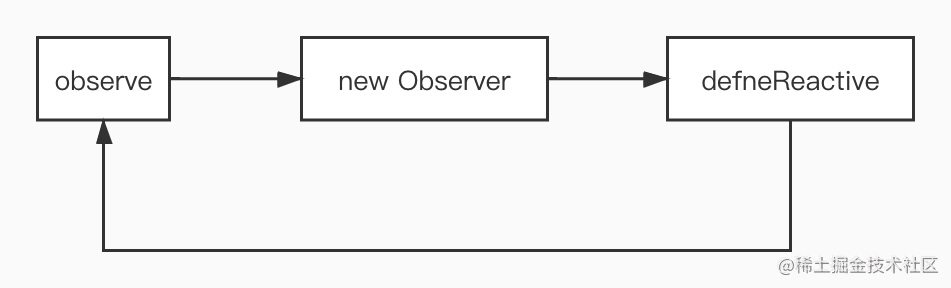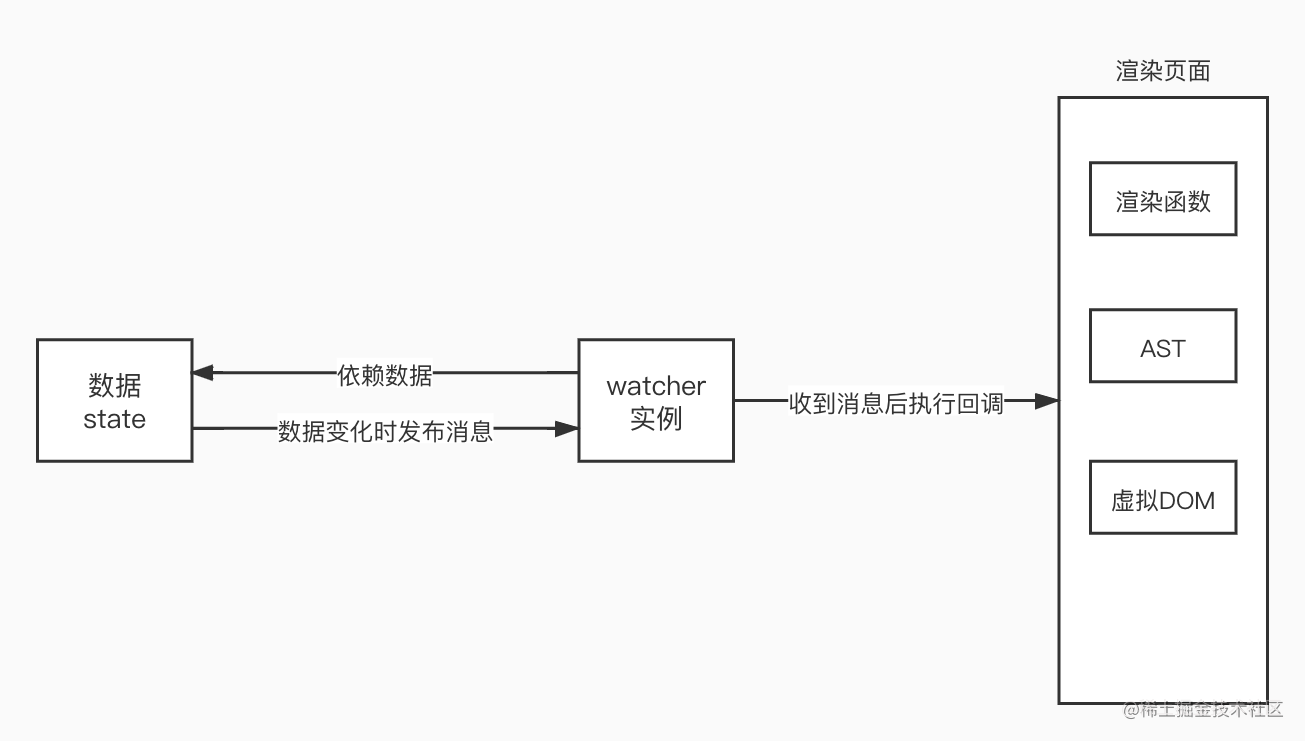# 0年前端的Vue响应式原理学习总结1：基本原理

2：数组的处理

3：渲染watcher

4：最终章

## 0.前言

1. 数据劫持：当数据变化时，我们可以做一些特定的事情
2. 依赖收集：我们要知道那些视图层的内容(`DOM`)依赖了哪些数据(`state`)
3. 派发更新：数据变化后，如何通知依赖这些数据的`DOM` 接下来，我们将一步步地实现一个自己的玩具响应式系统

## 1. 数据劫持

`Object.defineProperty`的用法在此不多做介绍，不明白的同学可在MDN上查阅。下面，我们为`obj`定义一个`a`属性

``````const obj = {}

let val = 1
Object.defineProperty(obj, a, {
get() { // 下文中该方法统称为getter
console.log('get property a')
return val
},
set(newVal) { // 下文中该方法统称为setter
if (val === newVal) return
console.log(`set property a -> \${newVal}`)
val = newVal
}
})

``````// value使用了参数默认值
function defineReactive(data, key, value = data[key]) {
Object.defineProperty(data, key, {
get: function reactiveGetter() {
return value
},
set: function reactiveSetter(newValue) {
if (newValue === value) return
value = newValue
}
})
}

defineReactive(obj, a, 1)

``````class Observer {
constructor(value) {
this.value = value
this.walk()
}
walk() {
Object.keys(this.value).forEach((key) => defineReactive(this.value, key))
}
}

const obj = { a: 1, b: 2 }
new Observer(obj)

``````// 入口函数
function observe(data) {
if (typeof data !== 'object') return
// 调用Observer
new Observer(data)
}

class Observer {
constructor(value) {
this.value = value
this.walk()
}
walk() {
// 遍历该对象，并进行数据劫持
Object.keys(this.value).forEach((key) => defineReactive(this.value, key))
}
}

function defineReactive(data, key, value = data[key]) {
// 如果value是对象，递归调用observe来监测该对象
// 如果value不是对象，observe函数会直接返回
observe(value)
Object.defineProperty(data, key, {
get: function reactiveGetter() {
return value
},
set: function reactiveSetter(newValue) {
if (newValue === value) return
value = newValue
observe(newValue) // 设置的新值也要被监听
}
})
}

const obj = {
a: 1,
b: {
c: 2
}
}

observe(obj)

``````执行observe(obj)
├── new Observer(obj),并执行this.walk()遍历obj的属性，执行defineReactive()
├── defineReactive(obj, a)
├── 执行observe(obj.a) 发现obj.a不是对象，直接返回
├── 执行defineReactive(obj, a) 的剩余代码
├── defineReactive(obj, b)
├── 执行observe(obj.b) 发现obj.b是对象
├── 执行 new Observer(obj.b)，遍历obj.b的属性，执行defineReactive()
├── 执行defineReactive(obj.b, c)
├── 执行observe(obj.b.c) 发现obj.b.c不是对象，直接返回
├── 执行defineReactive(obj.b, c)的剩余代码
├── 执行defineReactive(obj, b)的剩余代码## 2. 收集依赖与派发更新

### 依赖

`Vue`响应式系统中，显卡对应数据，那么例子中的买家对应什么呢？就是一个抽象的类: `Watcher`。大家不必纠结这个名字的含义，只需要知道它做什么事情：每个`Watcher`实例订阅一个或者多个数据，这些数据也被称为`wacther`的依赖(商品就是买家的依赖)；当依赖发生变化，`Watcher`实例会接收到数据发生变化这条消息，之后会执行一个回调函数来实现某些功能，比如更新页面(买家进行一些动作)。``````class Watcher {
constructor(data, expression, cb) {
// data: 数据对象，如obj
// expression：表达式，如b.c，根据data和expression就可以获取watcher依赖的数据
// cb：依赖变化时触发的回调
this.data = data
this.expression = expression
this.cb = cb
// 初始化watcher实例时订阅数据
this.value = this.get()
}

get() {
const value = parsePath(this.data, this.expression)
return value
}

// 当收到数据变化的消息时执行该方法，从而调用cb
update() {
this.value = parsePath(this.data, this.expression) // 对存储的数据进行更新
cb()
}
}

function parsePath(obj, expression) {
const segments = expression.split('.')
for (let key of segments) {
if (!obj) return
obj = obj[key]
}
return obj
}

1. 有一个数组来存储`watcher`
2. `watcher`实例需要订阅(依赖)数据，也就是获取依赖或者收集依赖
3. `watcher`的依赖发生变化时触发`watcher`的回调函数，也就是派发更新。

``````function defineReactive(data, key, value = data[key]) {
const dep = [] // 增加
observe(value)
Object.defineProperty(data, key, {
get: function reactiveGetter() {
return value
},
set: function reactiveSetter(newValue) {
if (newValue === value) return
value = newValue
observe(newValue)
dep.notify()
}
})
}

### 依赖收集

`new Watcher()`时执行`constructor`，调用了实例的`get`方法，实例的`get`方法会读取数据的值，从而触发了数据的`getter``getter`执行完毕后，实例的`get`方法执行完毕，并返回值，`constructor`执行完毕，实例化完毕。

``````get: function reactiveGetter() {
dep.push(watcher) // 新增
return value
}

``````get() {
window.target = this // 新增
const value = parsePath(this.data, this.expression)
return value
}

### 派发更新

``````set: function reactiveSetter(newValue) {
if (newValue === value) return
value = newValue
observe(newValue)
dep.forEach(d => d.update()) // 新增 update方法见Watcher类
}

## 3. 优化代码

### 1. Dep类

``````class Dep {
constructor() {
this.subs = []
}

depend() {
}

notify() {
const subs = [...this.subs]
subs.forEach((s) => s.update())
}

this.subs.push(sub)
}
}

`defineReactive`函数只需做相应的修改

``````function defineReactive(data, key, value = data[key]) {
const dep = new Dep() // 修改
observe(value)
Object.defineProperty(data, key, {
get: function reactiveGetter() {
dep.depend() // 修改
return value
},
set: function reactiveSetter(newValue) {
if (newValue === value) return
value = newValue
observe(newValue)
dep.notify() // 修改
}
})
}

### 2. window.target

`watcher``get`方法中

``````get() {
window.target = this // 设置了window.target
const value = parsePath(this.data, this.expression)
return value
}

``````// Watcher的get方法
get() {
window.target = this
const value = parsePath(this.data, this.expression)
window.target = null // 新增，求值完毕后重置window.target
return value
}

// Dep的depend方法
depend() {
if (Dep.target) { // 新增
}
}

### 3. update方法

``````update() {
this.value = parsePath(this.data, this.expression)
this.cb()
}

``````update() {
const oldValue = this.value
this.value = parsePath(this.data, this.expression)
this.cb.call(this.data, this.value, oldValue)
}

### 4. 学习一下Vue源码

Vue源码--56行中，我们会看到这样一个变量：`targetStack`，看起来好像和我们的`window.target`有点关系，没错，确实有关系。设想一个这样的场景：我们有两个嵌套的父子组件，渲染父组件时会新建一个父组件的`watcher`，渲染过程中发现还有子组件，就会开始渲染子组件，也会新建一个子组件的`watcher`。在我们的实现中，新建父组件`watcher`时，`window.target`会指向父组件`watcher`，之后新建子组件`watcher``window.target`将被子组件`watcher`覆盖，子组件渲染完毕，回到父组件`watcher`时，`window.target`变成了`null`，这就会出现问题，因此，我们用一个栈结构来保存`watcher`

``````const targetStack = []

function pushTarget(_target) {
targetStack.push(window.target)
window.target = _target
}

function popTarget() {
window.target = targetStack.pop()
}

`Watcher``get`方法做如下修改

``````get() {
pushTarget(this) // 修改
const value = parsePath(this.data, this.expression)
popTarget() // 修改
return value
}

### 5.总结代码

``````// 调用该方法来检测数据
function observe(data) {
if (typeof data !== 'object') return
new Observer(data)
}

class Observer {
constructor(value) {
this.value = value
this.walk()
}
walk() {
Object.keys(this.value).forEach((key) => defineReactive(this.value, key))
}
}

// 数据拦截
function defineReactive(data, key, value = data[key]) {
const dep = new Dep()
observe(value)
Object.defineProperty(data, key, {
get: function reactiveGetter() {
dep.depend()
return value
},
set: function reactiveSetter(newValue) {
if (newValue === value) return
value = newValue
observe(newValue)
dep.notify()
}
})
}

// 依赖
class Dep {
constructor() {
this.subs = []
}

depend() {
if (Dep.target) {
}
}

notify() {
const subs = [...this.subs]
subs.forEach((s) => s.update())
}

this.subs.push(sub)
}
}

Dep.target = null

const TargetStack = []

function pushTarget(_target) {
TargetStack.push(Dep.target)
Dep.target = _target
}

function popTarget() {
Dep.target = TargetStack.pop()
}

// watcher
class Watcher {
constructor(data, expression, cb) {
this.data = data
this.expression = expression
this.cb = cb
this.value = this.get()
}

get() {
pushTarget(this)
const value = parsePath(this.data, this.expression)
popTarget()
return value
}

update() {
const oldValue = this.value
this.value = parsePath(this.data, this.expression)
this.cb.call(this.data, this.value, oldValue)
}
}

// 工具函数
function parsePath(obj, expression) {
const segments = expression.split('.')
for (let key of segments) {
if (!obj) return
obj = obj[key]
}
return obj
}

// for test
let obj = {
a: 1,
b: {
m: {
n: 4
}
}
}

observe(obj)

let w1 = new Watcher(obj, 'a', (val, oldVal) => {
console.log(`obj.a 从 \${oldVal}(oldVal) 变成了 \${val}(newVal)`)
})

## 4. 注意事项

### 1. 闭包

`Vue`能够实现如此强大的功能，离不开闭包的功劳：在`defineReactive`中就形成了闭包，这样每个对象的每个属性就能保存自己的值`value`和依赖对象`dep`

### 3. 依赖嵌套的对象属性

``````let w2 = new Watcher(obj, 'b.m.n', (val, oldVal) => {
console.log(`obj.b.m.n 从 \${oldVal}(oldVal) 变成了 \${val}(newVal)`)
})

``````function parsePath(obj, expression) {
const segments = expression.split('.') // 先将表达式分割，segments:['b', 'm', 'n']
// 循环取值
for (let key of segments) {
if (!obj) return
obj = obj[key]
}
return obj
}

1. 局部变量`obj`为对象`obj`，读取`obj.b`的值，触发`getter`，触发`dep.depend()`(该`dep``obj.b`的闭包中的`dep`)，`Dep.target`存在，添加依赖
2. 局部变量`obj``obj.b`，读取`obj.b.m`的值，触发`getter`，触发`dep.depend()`(该`dep``obj.b.m`的闭包中的`dep`)，`Dep.target`存在，添加依赖
3. 局部变量`obj`为对象`obj.b.m`，读取`obj.b.m.n`的值，触发`getter`，触发`dep.depend()`(该`dep``obj.b.m.n`的闭包中的`dep`)，`Dep.target`存在，添加依赖

## 5. 总结

1. 调用`observe(obj)`，将`obj`设置为响应式对象，`observe函数，Observe, defineReactive函数`三者互相调用，从而递归地将`obj`设置为响应式对象
2. 渲染页面时实例化`watcher`，这个过程会读取依赖数据的值，从而完成`在getter中获取依赖`
3. 依赖变化时触发`setter`，从而派发更新，执行回调，完成`在setter中派发更新`